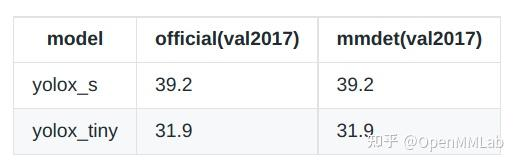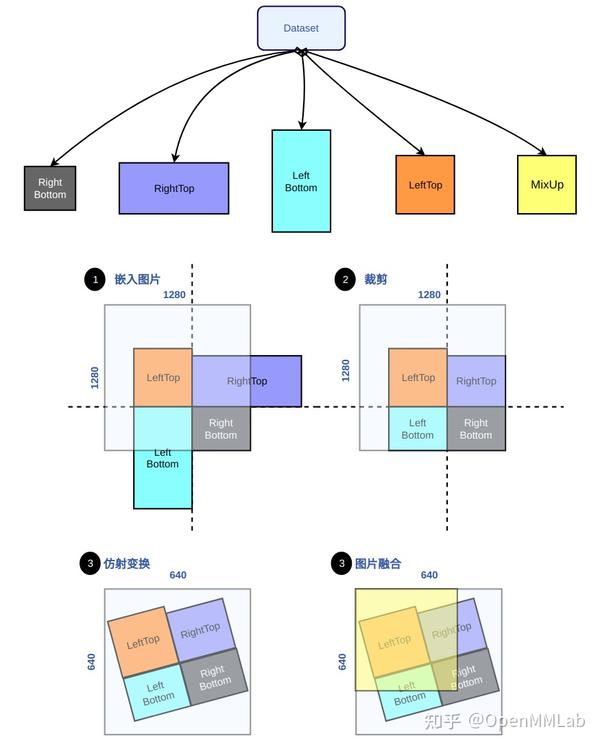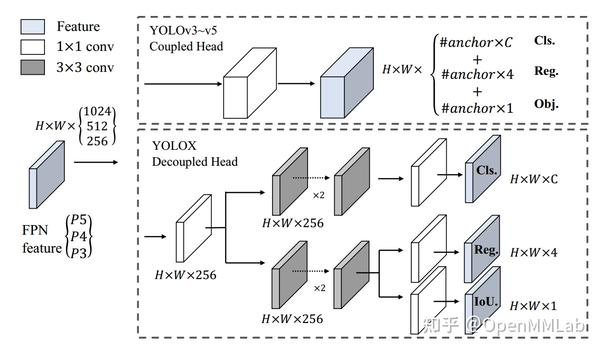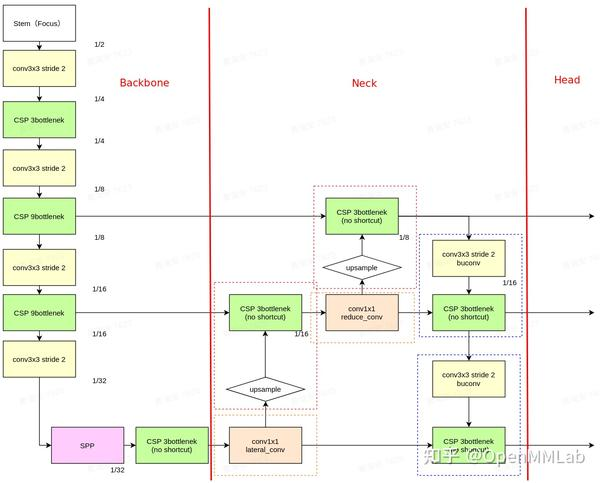• 问答
• 技术
• 实践
• 资源
YOLOX 在 MMDetection 中复现全流程解析

200条CV人的技术留言，学习路线/避坑指南（下拉 加载更多查看）：https://bbs.cvmart.net/articles/5369

1 YOLOX 算法简介

MMDetection 开源地址：GitHub - open-mmlab/mmdetection: OpenMMLab Detection Toolbox and Benchmark，欢迎 star

YOLOX 的主要特性可以归纳为：

1. Anchor-free，无需设计 anchor，更少先验，减少复杂超参，推理较高效
2. 提出了 Decoupled Head，参考 FCOS 算法设计解耦了分类和回归分支，同时新增 objectness 回归分支
3. 为了加快收敛以及提高性能，引入了 SimOTA 标签分配策略，其包括两部分 Multi positives 和 SimOTA，Multi positives 可以简单的增加每个 gt 所需的正样本数，SimOTA 基于 CVPR2021 最新的 OTA 算法，采用最优传输理论全局分配每个 gt 的正样本，考虑到 OTA 带来的额外训练代价，作者提出了简化版本的 OTA ，在长 epoch 训练任务中 SimOTA 和 OTA 性能相当，且可以极大的缩小训练代价
4. 参考 YOLOV4 的数据增强策略，引入了 Mosaic 和 MixUp，并且在最后 15 个 epoch 时候关闭这两个数据增强操作，实验表明可以极大地提升性能
5. 基于上述实践，参考 YOLOV5 网络设计思路，提出了 YOLOX-Nano、YOLOX-Tiny、YOLOX-S、YOLOX-M、YOLOX-L 和 YOLOX-X 不同参数量的模型

2 YOLOX 复现流程全解析

1. 推理精度对齐
2. 训练精度对齐
3. 重构

2.1 推理精度对齐

# 前处理核心操作
def preproc(image, input_size, mean, std, swap=(2, 0, 1)):
# 预定义输出图片大小
padded_img = np.ones((input_size, input_size, 3)) * 114.0

# 保持宽高比的 resize
img = np.array(image)
r = min(input_size / img.shape, input_size / img.shape)
resized_img = cv2.resize(
img,
(int(img.shape * r), int(img.shape * r)),
interpolation=cv2.INTER_LINEAR,
).astype(np.float32)

padded_img[: int(img.shape * r), : int(img.shape * r)] = resized_img

# bgr -> rgb

# 减均值，除方差
if mean is not None:
if std is not None:

test_pipeline = [
dict(
type='MultiScaleFlipAug',
img_scale=img_scale,
flip=False,
transforms=[
dict(type='Resize', keep_ratio=True),
dict(type='RandomFlip'),
dict(type='Normalize', **img_norm_cfg),
dict(type='DefaultFormatBundle'),
dict(type='Collect', keys=['img'])
])
]

test_cfg=dict(
score_thr=0.001,
nms=dict(type='nms', iou_threshold=0.65)))2.2 训练精度对齐

1. 优化器和学习率调度器
2. EMA 策略
3. Dataset
4. Loss
5. 其他训练 trick

2.2.1 优化器和学习率调度器

(1) 优化器

optimizer = dict(
type='SGD',
lr=0.01,
momentum=0.9,
weight_decay=5e-4,
nesterov=True,
paramwise_cfg=dict(norm_decay_mult=0., bias_decay_mult=0.))

(2) 学习率调度器

YOLOX 的学习率调度器是带有 warmup 策略的余弦调度策略，并且为了配合数据增强，在最后 15 个 epoch 会采用固定的最小学习率

MMDetection 中导入的 MMCV 已经实现了带有 warmup 策略的余弦调度策略，但是比较麻烦的是指数 warmup 策略公式不太一样，并且不具有在最后 15 个 epoch 采用固定最小学习率的功能。为了解决上述问题，且不更改依赖 MMCV 版本，我们是在 MMDetection 中继承了原先的 CosineAnnealingLrUpdaterHook，并重写了相关方法。

class YOLOXLrUpdaterHook(CosineAnnealingLrUpdaterHook):
def get_warmup_lr(self, cur_iters):

def _get_warmup_lr(cur_iters, regular_lr):
# 重写 warmup 策略
k = self.warmup_ratio * pow(
(cur_iters + 1) / float(self.warmup_iters), 2)
warmup_lr = [_lr * k for _lr in regular_lr]
return warmup_lr

...

def get_lr(self, runner, base_lr):

progress = runner.iter
max_progress = runner.max_iters

progress += 1

target_lr = base_lr * self.min_lr_ratio

if progress >= max_progress - last_iter:
# 固定学习率策略
return target_lr
else
return annealing_cos(
base_lr, target_lr, (progress - self.warmup_iters) /
(max_progress - self.warmup_iters - last_iter))

2.2.2 EMA 策略

def update(self, model):
# Update EMA parameters
# self.decay = lambda x: decay * (1 - math.exp(-x / 2000))

msd = (
model.module.state_dict() if is_parallel(model) else model.state_dict()
)
for k, v in self.ema.state_dict().items():
if v.dtype.is_floating_point:
v *= d
v += (1.0 - d) * msd[k].detach()

MMCV 中已经通过 Hook 实现了 EMA 功能，但是没有考虑 BN buffer 的 EMA 操作，而且 decay 公式也不一样，MMCV 中是线性 decay 策略，而 YOLOX 中是指数 decay。为此，我们在兼容 MMCV EMA 功能的前提下，重新设计了 EMA Hook，目前暂时放在 MMDetection 中，会在后续版本迁移到 MMCV 中。

EMA 的实现是采用 Hook 实现的，下面贴核心代码：

@HOOKS.register_module()
class EMAHook(Hook):
# 在模型运行前，将模型参数重新 copy 一份，然后重新作为 buffer 插入到模型中
# 也就是说此时 Model 里面有两份相同参数的模型了
def before_run(self, runner):
model = runner.model
if is_module_wrapper(model):
model = model.module
self.param_ema_buffer = {}
self.model_parameters = dict(model.named_parameters(recurse=True))
for name, value in self.model_parameters.items():
# "." is not allowed in module's buffer name
buffer_name = f"ema_{name.replace('.', '_')}"
self.param_ema_buffer[name] = buffer_name
model.register_buffer(buffer_name, value.data.clone())
self.model_buffers = dict(model.named_buffers(recurse=True))
# 这个很关键，后续会说明
if self.checkpoint is not None:
runner.resume(self.checkpoint)

# 实时计算更新 ema 模型参数
def after_train_iter(self, runner):
curr_step = runner.iter
# We warm up the momentum considering the instability at beginning
momentum = min(self.momentum,
(1 + curr_step) / (self.warm_up + curr_step))
if curr_step % self.interval != 0:
return
for name, parameter in self.model_parameters.items():
buffer_name = self.param_ema_buffer[name]
buffer_parameter = self.model_buffers[buffer_name]
# 非常关键，后续说明
def after_train_epoch(self, runner):
"""We load parameter values from ema backup to model before the
EvalHook."""
self._swap_ema_parameters()

def before_train_epoch(self, runner):
"""We recover model's parameter from ema backup after last epoch's
EvalHook."""
self._swap_ema_parameters()

def _swap_ema_parameters(self):
"""Swap the parameter of model with parameter in ema_buffer."""
for name, value in self.model_parameters.items():
temp = value.data.clone()
ema_buffer = self.model_buffers[self.param_ema_buffer[name]]
value.data.copy_(ema_buffer.data)
ema_buffer.data.copy_(temp)

1. 首先在外面构建模型参数和初始化模型
2. 在 before_run 中新建一份 ema 模型参数的 buffer，并且插入到原先模型中
3. 在开启 epoch 训练前 before_train_epoch 交换一次权重，即 model 的参数值是 ema 参数值，而 ema 参数值是 model，并且由于此时两者完全相等，所以可以认为没有交换
4. 迭代的更新 model 参数，并且在 after_train_iter 中实时更新 ema 模型参数
5. 由于 EMAHook 优先级较高，故会优先于 evalhook 和 save checkpoint 相关逻辑，其会先运行 after_train_epoch 交换一次参数，此时 model 的参数值是 ema 参数值，而 ema 参数值是 model，完成了一次真正的参数交换
6. 运行 evalhook 和 save checkpoint 相关逻辑，其面对的 model 是 ema model，符合预期
7. 在下一次循环迭代时候，重复 3-6 过程，不断的交换参数

1. 首先在外面构建模型参数和初始化模型
2. resume 模型时候，由于此时 ema 模型还没有构建，所以只能加载权重字典中的 model 字段，但实际上该 model 字段是 ema
3. 在 before_run 中新建一份 ema 模型参数的 buffer，并且插入到原先模型中，此时就构建出了 ema 模型
4. 如果传入了待 resume 的 checkpoint，此时会重新加载一遍，由于 ema 模型已经构建，所以 ema 模型和 model 都会被 resume，同时 ema 模型参数是 model，而 model 参数是 ema
5. 在开启 epoch 训练前 before_train_epoch 交换一次权重，此时就正确了，也就是说到这一步就完全恢复了
6. 继续正常的训练

(1) 考虑要能够平滑 BN 的 buffer 参数，我们增加了 skip_buffers 参数

if self.skip_buffers:
# 如果跳过，那就直接用参数即可
self.model_parameters = dict(model.named_parameters())
else:
# 如果不跳过，则直接使用状态字典
self.model_parameters = model.state_dict()

(2) 考虑会可能存在多种平滑曲线，我们设计了 BaseEMAHook，然后继承这个类进行扩展不同的平滑曲线

@HOOKS.register_module()
class ExpMomentumEMAHook(BaseEMAHook):

def __init__(self, total_iter=2000, **kwargs):
super(ExpMomentumEMAHook, self).__init__(**kwargs)
self.momentum_fun = lambda x: (1 - self.momentum) * math.exp(-(
1 + x) / total_iter) + self.momentum

@HOOKS.register_module()
class LinearMomentumEMAHook(BaseEMAHook):

def __init__(self, warm_up=100, **kwargs):
super(LinearMomentumEMAHook, self).__init__(**kwargs)
self.momentum_fun = lambda x: min(self.momentum**self.interval,
(1 + x) / (warm_up + x))

2.2.3 Dataset

dataset 部分最复杂，其包括 Mosaic 、MixUp、ColorJit 和动态 resize 等等操作。由于 MMDetection 中暂时都没有实现上述组件，并且超参很多，为此在我们第一版中实际上是直接复制了源码的 dataset，只对 dataset 输出进行包装，使其能够接入 MMDetection 训练过程，在训练精度对齐后进行重新设计。本小结先简要分析源码，然后再描述 MMDetection 实现过程。

(1) 源码解释

dataset = COCODataset(...)

dataset = MosaicDetection(
dataset,
mosaic=not no_aug,
img_size=self.input_size,
preproc=TrainTransform(
rgb_means=(0.485, 0.456, 0.406),
std=(0.229, 0.224, 0.225),
max_labels=120,
),
degrees=self.degrees,
translate=self.translate,
scale=self.scale,
shear=self.shear,
perspective=self.perspective,
enable_mixup=self.enable_mixup,
)

def __getitem__(self, idx):
# 是否进入 mosaic，默认前 285 个 epoch 都会进入
if self.enable_mosaic:
1 马赛克增强
2 几何变换增强
# 是否进入 mixup，nano 和 tiny 版本默认是关闭的
if self.enable_mixup and not len(mosaic_labels) == 0:
3 mixup 增强
4 图片后处理
else：
4 图片后处理1) 马赛克增强

1. 随机出 4 张图片在待输出图片中交接的中心点坐标
2. 随机出另外 3 张图片的索引以及读取对应的标注
3. 对每张图片采用保持宽高比的 resize 操作缩放到指定大小
4. 按照上下左右规则，计算每张图片在待输出图片中应该放置的位置，因为图片可能出界故还需要计算裁剪坐标
5. 利用裁剪坐标将缩放后的图片裁剪，然后贴到前面计算出的位置，其余位置全部补 114 像素值
6. 对每张图片的标注也进行相应处理
7. 由于拼接了 4 张图，所以输出图片大小会扩大 4 倍

2) 几何变换增强

random_perspective 包括平移、旋转、缩放、错切等增强，并且会将输入图片还原为 (640, 640)，同时对增强后的标注进行处理，过滤规则是

1. 增强后的 gt bbox 宽高要大于 wh_thr
2. 增强后的 gt bbox 面积和增强前的 gt bbox 面积要大于 ar_thr，防止增强太严重
3. 最大宽高比要小于 area_thr，防止宽高比改变太多

3) MixUp

Mixup 实现方法有多种，常见的做法是：要么 label 直接拼接起来，要么 label 也采用 alpha 混合，作者的做法非常简单，对 label 直接拼接即可，而图片也是采用固定的 0.5:0.5 混合方法。

1. 随机出一张图片，必须要保证该图片不是空标注
2. 对随机出的图片采用保持宽高比的 resize 操作缩放到指定大小
5. 随机采用 flip 增强
6. 如果处理后的图片比原图大，则还需要进行随机裁剪增强
7. 对标签进行对应处理，并且采用和马赛克增强一样的过滤规则
8. 如果过滤后还存在 gt bbox，则采用 0.5:0.5 的比例混合原图和处理后的图片，标签则直接拼接即可

4) 图片后处理

1. 随机 ColorJit，包括众多颜色相关增强
2. 随机翻转增强
3. 对随机后的图片采用保持宽高比的 resize 操作缩放到指定大小
4. 对于宽高小于 8 像素的 gt bbox 直接删掉，因为网络输出的最小 stride 是 8

(2) MMDetection 实现

Dataset 部分涉及到的代码比较多，主要包括：

1. 之前框架中还没有实现过类似 Mosaic 等需要再次利用 dataset 相关信息的代码
2. 之前框架中还没有实现过类似在某一阶段关闭某些数据增强的操作

• 类似 RandomFlip 等，作为 pipeline 实现

mosaic_pipeline = [
dict(type='PhotoMetricDistortion'),
dict(
type='Expand',
mean=img_norm_cfg['mean'],
to_rgb=img_norm_cfg['to_rgb'],
ratio_range=(1, 2)),
]

mosaic_data = dict(
type=dataset_type,
ann_file=data_root + 'annotations/instances_train2017.json',
img_prefix=data_root + 'train2017/',
pipeline=mosaic_pipeline)

train_pipeline = [
dict(type='PhotoMetricDistortion'),
dict(
type='Expand',
mean=img_norm_cfg['mean'],
to_rgb=img_norm_cfg['to_rgb'],
ratio_range=(1, 2)),
dict(type='Mosaic', size=(416, 416), dataset=mosaic_data, min_offset=0.2),
dict(type='Resize', img_scale=[(320, 320), (416, 416)], keep_ratio=True),
dict(type='RandomFlip', flip_ratio=0.5),
dict(type='Normalize', **img_norm_cfg),
dict(type='DefaultFormatBundle'),
dict(type='Collect', keys=['img', 'gt_bboxes', 'gt_labels'])
]

• 类似 RandomFlip 等，作为 pipeline 实现，但是内部缓存 index

• 类似 RepeatDataset 等，作为 dataset wrapper 实现

def __getitem__(self, idx):
# 获取当前 idx 的图片信息
results = self.dataset[idx]
# 遍历 transorm,其中可以包括 mosaic 、mixup、flip 等各种  transform
for (transform, transform_type) in zip(self.pipeline,
self.pipeline_types):
# 考虑到某些训练阶段需要动态关闭掉部分数据增强，故引入   _skip_type_keys
if self._skip_type_keys is not None and \
transform_type in self._skip_type_keys:
continue
if hasattr(transform, 'get_indexes'):
# transform 如果额外提供了 get_indexes 方法，则表示需要进行混合数据增强
# 返回索引
indexes = transform.get_indexes(self.dataset)
if not isinstance(indexes, collections.abc.Sequence):
indexes = [indexes]
# 得到混合图片信息
mix_results = [
copy.deepcopy(self.dataset[index]) for index in indexes
]
results['mix_results'] = mix_results
# 动态尺度 resize
if self._dynamic_scale is not None:
# Used for subsequent pipeline to automatically change
# the output image size. E.g MixUp, Resize.
results['scale'] = self._dynamic_scale
# 数据增强
results = transform(results)

if 'mix_results' in results:
results.pop('mix_results')
if 'img_scale' in results:
results.pop('img_scale')
return results

@PIPELINES.register_module()
class Mosaic:
def __init__(self,
img_scale=(640, 640),
center_ratio_range=(0.5, 1.5),
assert isinstance(img_scale, tuple)
self.img_scale = img_scale
self.center_ratio_range = center_ratio_range

def __call__(self, results):
results = self._mosaic_transform(results)
return results

def get_indexes(self, dataset):
indexs = [random.randint(0, len(dataset)) for _ in range(3)]
return indexs

@PIPELINES.register_module()
class MixUp:
def __init__(self,
img_scale=(640, 640),
ratio_range=(0.5, 1.5),
flip_ratio=0.5,
max_iters=15,
min_bbox_size=5,
min_area_ratio=0.2,
max_aspect_ratio=20):
assert isinstance(img_scale, tuple)
self.dynamic_scale = img_scale
self.ratio_range = ratio_range
self.flip_ratio = flip_ratio
self.max_iters = max_iters
self.min_bbox_size = min_bbox_size
self.min_area_ratio = min_area_ratio
self.max_aspect_ratio = max_aspect_ratio

def __call__(self, results):
results = self._mixup_transform(results)
return results

# 必须要返回非空 gt bbox 数据索引
def get_indexes(self, dataset):
for i in range(self.max_iters):
index = random.randint(0, len(dataset))
gt_bboxes_i = dataset.get_ann_info(index)['bboxes']
if len(gt_bboxes_i) != 0:
break

return index

train_pipeline = [
dict(
type='RandomAffine',
scaling_ratio_range=(0.1, 2),
border=(-img_scale // 2, -img_scale // 2)),
dict(type='MixUp', img_scale=img_scale, ratio_range=(0.8, 1.6)),
# PhotoMetricDistortion 和 YOLOX 中实现的颜色增强不一样，PhotoMetricDistortion 增强更强一些，但是考虑随机操作可能对最终性能没有很大影响，故我们并没有对其进行修改
dict(
type='PhotoMetricDistortion',
brightness_delta=32,
contrast_range=(0.5, 1.5),
saturation_range=(0.5, 1.5),
hue_delta=18),
dict(type='RandomFlip', flip_ratio=0.5),
dict(type='Resize', keep_ratio=True),
dict(type='Normalize', **img_norm_cfg),
dict(type='DefaultFormatBundle'),
dict(type='Collect', keys=['img', 'gt_bboxes', 'gt_labels'])
]

train_dataset = dict(
type='MultiImageMixDataset',
dataset=dict(
type=dataset_type,
ann_file=data_root + 'annotations/instances_train2017.json',
img_prefix=data_root + 'train2017/',
pipeline=[
],
filter_empty_gt=False,
),
pipeline=train_pipeline,
dynamic_scale=img_scale)

2.2.4 Loss

(1) 源码解释

1) 计算 3 个输出层所需要的特征图尺度的坐标，用于 bbox 解码

yv, xv = torch.meshgrid([torch.arange(hsize), torch.arange(wsize)])
grid = torch.stack((xv, yv), 2).view(1, 1, hsize, wsize, 2).type(dtype)

2) 对输出 bbox 进行解码还原到原图尺度

output = output.view(batch_size, 1, n_ch, hsize, wsize)
output = output.permute(0, 1, 3, 4, 2).reshape(
batch_size, 1 * hsize * wsize, -1
)
grid = grid.view(1, -1, 2)
# 还原
output[..., :2] = (output[..., :2] + grid) * stride
output[..., 2:4] = torch.exp(output[..., 2:4]) * stride

3) 对每张图片单独计算匹配的正样本和对应的 target

1. 基于 grid 和 stride 计算 anchor 点的中心坐标，其中 anchor 是个数为 1 的正方形 anchor
2. 计算所有 gt bbox 的中心坐标
3. 计算所有在 gt bbox 内部的 anchor 点的掩码 is_in_boxes_all
4. 利用 center_radius 阈值重新计算在 gt bbox 中心 center_radius 范围内的 anchor 点的掩码 is_in_centers_all
5. 两个掩码取并集得到在 gt bbox 内部或处于 center_radius 范围内的 anchor 点的掩码 is_in_boxes_anchor，同时可以取交集得到每个 gt bbox 和哪些 anchor 点符合 gt bbox 内部和处于 center_radius 范围内的 anchor is_in_boxes_and_center

4) 计算每张图片中 gt bbox 和候选预测框的匹配代价

# fg_mask : (n,) 如果某个位置是 True 代表该 anchor 点是前景即
# 落在 gt bbox 内部或者在距离 gt bbox 中心 center_radius 半径范围内
# is_in_boxes_and_center：(num_gt,n), 如果某个位置是 True 代表
# 该 anchor 点落在 gt bbox 内部并且在距离 gt bbox 中心 center_radius 半径范围内
# 提取对应值
num_in_boxes_anchor = bboxes_preds_per_image.shape

# 计算预测框和 gt bbox 的配对 iou
pair_wise_ious = bboxes_iou(gt_bboxes_per_image, bboxes_preds_per_image, False)
gt_cls_per_image = (
F.one_hot(gt_classes.to(torch.int64), self.num_classes)
.float()
.unsqueeze(1)
.repeat(1, num_in_boxes_anchor, 1)
)
# iou 越大，匹配度越高，所以需要取负号
pair_wise_ious_loss = -torch.log(pair_wise_ious + 1e-8)

cls_preds_ = (
cls_preds_.float().unsqueeze(0).repeat(num_gt, 1, 1).sigmoid_()
* obj_preds_.unsqueeze(0).repeat(num_gt, 1, 1).sigmoid_()
)
# 配对的分类 Loss，包括了 iou 分支预测值
pair_wise_cls_loss = F.binary_cross_entropy(
cls_preds_.sqrt_(), gt_cls_per_image, reduction="none"
).sum(-1)
del cls_preds_

# 计算每个 gt bbox 和选择出来的候选预测框的分类 loss + 坐标 loss + 中心点和半径约束
# 值越小，表示匹配度越高
# (num_gt,n)
cost = (
pair_wise_cls_loss
+ 3.0 * pair_wise_ious_loss
+ 100000.0 * (~is_in_boxes_and_center)
)
1. fg_mask 就是前面计算出的 is_in_boxes_anchor，如果某个位置是 True 代表该 anchor 点是前景即落在 gt bbox 内部或者在距离 gt bbox 中心 center_radius 半径范围内，这些 True 位置就是正样本候选点
2. 利用 fg_mask 提取对应的预测信息，假设 num_gt 是 3，一共提取了 800 个候选预测位置，则每个 gt bbox 都会提取出 800 个候选位置
3. 计算候选预测框和 gt bbox 的配对 iou，然后加 log 和负数，变成 iou 的代价函数
4. 计算候选预测框和 gt bbox 的配对分类代价值，同时考虑了 objectness 预测分支，并且其分类 cost 在 binary_cross_entropy 前有开根号的训练 trick
5. is_in_boxes_and_center shape 是 (3, 800), 如果某个位置是 True 表示该 anchor 点落在 gt bbox 内部并且在距离 gt bbox 中心 center_radius 半径范围内。在计算代价函数时候，如果该预测点是 False，表示不再交集内部，那么应该不太可能是候选点，所以给予一个非常大的代价权重 100000.0，该操作可以保证每个 gt bbox 最终选择的候选点不会在交集外部

5) 为每个 gt bbox 动态选择 k 个 候选预测值，作为匹配正样本

def dynamic_k_matching(self, cost, pair_wise_ious, gt_classes, num_gt, fg_mask):
# 匹配矩阵初始化为 0
matching_matrix = torch.zeros_like(cost)
ious_in_boxes_matrix = pair_wise_ious
# 每个 gt bbox 选择的候选预测点不超过 10 个
n_candidate_k = min(10, ious_in_boxes_matrix.size(1))
topk_ious, _ = torch.topk(ious_in_boxes_matrix, n_candidate_k, dim=1)
# 每个 gt bbox 的动态 k
dynamic_ks = torch.clamp(topk_ious.sum(1).int(), min=1)
for gt_idx in range(num_gt):
_, pos_idx = torch.topk(
cost[gt_idx], k=dynamic_ks[gt_idx].item(), largest=False
)
# 匹配上位置设置为 1
matching_matrix[gt_idx][pos_idx] = 1.0
del topk_ious, dynamic_ks, pos_idx
# n, 表示该候选点有没有匹配到 gt bbox
anchor_matching_gt = matching_matrix.sum(0)
if (anchor_matching_gt > 1).sum() > 0:
# 如果某个候选点匹配了多个 gt bbox，则选择代价最小的，保证每个候选点只能匹配一个 gt bbox
_, cost_argmin = torch.min(cost[:, anchor_matching_gt > 1], dim=0)
matching_matrix[:, anchor_matching_gt > 1] *= 0.0
matching_matrix[cost_argmin, anchor_matching_gt > 1] = 1.0

# 每个候选点的匹配情况
# 总共有多少候选点
# 更新前景掩码，在前面中心先验的前提下进一步筛选正样本

# 该候选框匹配到哪个 gt bbox
gt_matched_classes = gt_classes[matched_gt_inds]

# 提取对应的预测点和gt bbox 的 iou
pred_ious_this_matching = (matching_matrix * pair_wise_ious).sum(0)[
]
return num_fg, gt_matched_classes, pred_ious_this_matching, matched_gt_inds
1. 初始化 gt bbox 和候选点的匹配矩阵为全 0，表示全部不匹配
2. 每个 gt bbox 选择的候选预测点不超过 10 个
3. 利用前面的匹配代价，给每个 gt bbox 计算动态 k
4. 遍历每个 gt bbox，提取代价为前动态 k 个位置，表示匹配上
5. 如果某个候选点匹配了多个 gt bbox，则选择代价最小的，保证每个候选点只能匹配一个 gt bbox
6. 返回总共有多少候选点 num_fg、每个候选点匹配上的 gt bbox 信息 gt_matched_classes、每个候选点匹配上的 和 gt bbox 计算的 IoU 值、匹配上的 gt bbox 索引、更新后的前景掩码 fg_mask，其长度和预测点个数相同，其中 1 表示正样本点，0 表示负样本点。

6) 计算 loss

1. 分类分支仅仅考虑正样本即 fg_mask 为 1 的位置，其 label 是同时考虑了预测值和 gt bbox 的 IoU 值，用于加强各分支间的一致性
2. objectness 分支需要同时考虑正负样本，其起到抑制背景的作用，其 label 就是上述 fg_mask，非 0 即 1，即所有候选点
3. bbox 分支也仅仅考虑正样本，其 label 就是正样本候选点所对应的解码后的预测值

7) 附加 L1 Loss

(2) MMDetection 重构版本

Loss 写法的重构，主要改动是采用 MMDetection 中默认的构建方式即 prior_generator + bbox assign + bbox encode decode + loss。

YOLOX 是 anchor-free 算法，但是依然需要特征图上每个预测点的 point 信息，为此我们直接采用 RepPoints 中的 MlvlPointGenerator 类用于生成 anchor-point 坐标；bbox assign 部分则重写并新建了 SimOTAAssigner 类，用于对预测点进行分配正负样本；其他地方则完善了命名规范，和简化了部分写法，使其更加容易理解，整体逻辑没有改动。

2.2.5 其他训练 trick

1. 在最后 15 个 epoch 关闭 Mosaic 和 MixUp 增强，并且增加额外的 L1 loss
2. 每隔一定间隔，改变输出图片尺寸，并且保证多卡之间的图片尺寸相同
3. 每隔一定间隔，对 BN 参数进行多卡同步，保证评估时候不同卡的权重性能一致

(1) 关闭 Mosaic 和 MixUp 增强，增加额外的 L1 loss

def before_epoch(self):

if self.epoch + 1 == self.max_epoch - self.exp.no_aug_epochs or self.no_aug:
logger.info("--->No mosaic aug now!")

MMDetection 是通过 hook 实现上述功能的

@HOOKS.register_module()
class YOLOXModeSwitchHook(Hook):

def __init__(self, num_last_epochs=15):
self.num_last_epochs = num_last_epochs

def before_train_epoch(self, runner):
"""Close mosaic and mixup augmentation and switches to use L1 loss."""
epoch = runner.epoch
model = runner.model
if is_parallel(model):
model = model.module
if (epoch + 1) == runner.max_epochs - self.num_last_epochs:
runner.logger.info('No mosaic and mixup aug now!')
['Mosaic', 'RandomAffine', 'MixUp'])

(2) 改变输出图片尺寸

# 在每次训练迭代后，判断
if self.exp.random_size is not None and (self.progress_in_iter + 1) % 10 == 0:
self.input_size = self.exp.random_resize(
)

def random_resize(self, data_loader, epoch, rank, is_distributed):
tensor = torch.LongTensor(2).cuda()

if rank == 0:
# 随机采样一个新的 size
size_factor = self.input_size * 1.0 / self.input_size
size = random.randint(*self.random_size)
size = (int(32 * size), 32 * int(size * size_factor))
tensor = size
tensor = size

if is_distributed:
dist.barrier()

# 改变图片 size
multiple=(tensor.item(), tensor.item()), random_range=None
)
return input_size

MMDetection 是通过 hook 实现上述功能的

@HOOKS.register_module()
class SyncRandomSizeHook(Hook):
"""Change and synchronize the random image size across ranks, currently
used in YOLOX.

Args:
ratio_range (tuple[int]): Random ratio range. It will be multiplied
by 32, and then change the dataset output image size.
Default: (14, 26).
img_scale (tuple[int]): Size of input image. Default: (640, 640).
interval (int): The interval of change image size. Default: 10.
"""

def __init__(self,
ratio_range=(14, 26),
img_scale=(640, 640),
interval=10):
self.rank, world_size = get_dist_info()
self.is_distributed = world_size > 1
self.ratio_range = ratio_range
self.img_scale = img_scale
self.interval = interval

def after_train_iter(self, runner):
"""Change the dataset output image size."""
if self.ratio_range is not None and (runner.iter +
1) % self.interval == 0:
# 同步动态 resize

(3) BN 参数进行多卡同步

def all_reduce_norm(module):
"""
All reduce norm statistics in different devices.
"""
states = get_async_norm_states(module)
states = all_reduce(states, op="mean")

MMDetection 是通过 hook 实现上述功能的，并且考虑多卡同步字典操作是通用的，为此将其封装到 get_norm_states(module) 中。

@HOOKS.register_module()
class SyncNormHook(Hook):

def __init__(self, interval=1):
self.interval = interval

def after_train_epoch(self, runner):
"""Synchronizing norm."""
epoch = runner.epoch
module = runner.model
if (epoch + 1) % self.interval == 0:
_, world_size = get_dist_info()
if world_size == 1:
return
norm_states = get_norm_states(module)
norm_states = all_reduce_dict(norm_states, op='mean')

(4) Hook 优先级注意事项

custom_hooks = [
dict(type='YOLOXModeSwitchHook', num_last_epochs=15, priority=48),
dict(
type='SyncRandomSizeHook',
ratio_range=(14, 26),
img_scale=img_scale,
interval=interval,
priority=48),
dict(type='SyncNormHook', interval=interval, priority=48),
dict(type='ExpMomentumEMAHook', resume_from=resume_from, priority=49)
]

evalhook 和保存权重操作的优先级是 50，优先级值越小优先级越高，为此我们将 ExpMomentumEMAHook 优先级调整为 49，其余三个 hook，优先级设置为 48 就行，这三个 hook 执行顺序没有特别要求，优先级相同则会依据插入顺序执行。

2.3 重构

(1) 模型相关重构1) 参考 ResNet 写法，arch_settings 按 stage 写，逐个 stage build

arch_settings = {
'P5': [[64, 128, 3, True, False],
[128, 256, 9, True, False],
[256, 512, 9, True, False],
[512, 1024, 3, False, True]],

'P6': [[64, 128, 3, True, False],
[128, 256, 9, True, False],
[256, 512, 9, True, False],
[512, 768, 3, True, False],
[768, 1024, 3, False, True]]
}

2) 参考 YOLOV5 写法，arch_settings 按 YOLOv5 config 的风格写，逐个 layer build

backbone:
# [from, number, module, args]
[ [ -1, 1, Focus, [ 64, 3 ] ],  # 0-P1/2
[ -1, 1, Conv, [ 128, 3, 2 ] ],  # 1-P2/4
[ -1, 3, C3, [ 128 ] ],
[ -1, 1, Conv, [ 256, 3, 2 ] ],  # 3-P3/8
[ -1, 9, C3, [ 256 ] ],
[ -1, 1, Conv, [ 512, 3, 2 ] ],  # 5-P4/16
[ -1, 9, C3, [ 512 ] ],
[ -1, 1, Conv, [ 768, 3, 2 ] ],  # 7-P5/32
[ -1, 3, C3, [ 768 ] ],
[ -1, 1, Conv, [ 1024, 3, 2 ] ],  # 9-P6/64
[ -1, 1, SPP, [ 1024, [ 3, 5, 7 ] ] ],
[ -1, 3, C3, [ 1024, False ] ],  # 11
]

@BACKBONES.register_module()
class CSPDarknet(BaseModule):

def __init__(self,
arch='P5',
...
init_cfg=dict(
type='Kaiming',
layer='Conv2d',
a=math.sqrt(5),
distribution='uniform',
mode='fan_in',
nonlinearity='leaky_relu')):

(2) 后处理相关重构

YOLOX 的后处理策略非常简单，只需要 conf_thr 和 nms 阈值就可以。

• 基于输出特征图宽高和 stride，生成 grid，然后基于 grid 对预测框进行解码还原到原图尺度
def decode_outputs(self, outputs, dtype):
grids = []
strides = []
for (hsize, wsize), stride in zip(self.hw, self.strides):
yv, xv = torch.meshgrid([torch.arange(hsize), torch.arange(wsize)])
grid = torch.stack((xv, yv), 2).view(1, -1, 2)
grids.append(grid)
shape = grid.shape[:2]
strides.append(torch.full((*shape, 1), stride))
grids = torch.cat(grids, dim=1).type(dtype)
strides = torch.cat(strides, dim=1).type(dtype)
# 解码还原
outputs[..., :2] = (outputs[..., :2] + grids) * strides
outputs[..., 2:4] = torch.exp(outputs[..., 2:4]) * strides

return outputs
• 利用 conf_thre 过滤 bbox
# 提取最大分值对应的类别和预测分值
class_conf, class_pred = torch.max(
image_pred[:, 5 : 5 + num_classes], 1, keepdim=True
)
# obj 分值和 class_conf 相乘，然后利用 conf_thre 进行过滤
conf_mask = (image_pred[:, 4] * class_conf.squeeze() >= conf_thre).squeeze()

# 拼接
detections = torch.cat((image_pred[:, :5], class_conf, class_pred.float()), 1)
• NMS 后处理
nms_out_index = torchvision.ops.batched_nms(
detections[:, :4],
detections[:, 4] * detections[:, 5],
detections[:, 6],
nms_thre,
)
detections = detections[nms_out_index]

MMDetection 后处理重构整体没有改变(因为已经很简单了)，只不过我们统一用 prior_generator 来生成 grid，其他地方统一了参数命名方式和代码风格。

3 总结

【极市开学礼，社区踩楼直接送】小米 11、海信电视（43英寸）、服务器、手环 5、200 京东卡（数张）、键鼠套装、漫步者头戴蓝牙耳机等 已准备就绪（下拉 加载更多查看）：https://bbs.cvmart.net/articles/5369

•3
•0
•5044收藏

• 18

关注
• 56

获赞
• 2

精选文章

• 目标检测

• shijie的专栏

• 2.9k
• 2.4k
• 5.0k
• 1.2k
• 2.7k